# Avoid Overfitting with Regularization

This article explains overfitting which is one of the reasons for poor predictions for unseen samples. Also, regularization technique based on regression is presented by simple steps to make it clear how to avoid overfitting.comments

Have you ever created a machine learning model that is perfect for the training samples but gives very bad predictions with unseen samples! Did you ever think why this happens? This article explains overfitting which is one of the reasons for poor predictions for unseen samples. Also, regularization technique based on regression is presented by simple steps to make it clear how to avoid overfitting.

The focus of machine learning (ML) is to train an algorithm with training data in order create a model that is able to make the correct predictions for unseen data (test data). To create a classifier, for example, a human expert will start by collecting the data required to train the ML algorithm. The human is responsible for finding the best types of features to represent each class which is capable of discriminating between the different classes. Such features will be used to train the ML algorithm. Suppose we are to build a ML model that classifies images as containing cats or not using the following training data.The first question we have to answer is “what are the best features to use?”. This is a critical question in ML as the better the used features the better the predictions the trained ML model makes and vice versa. Let us try to visualize such images and extract some features that are representative of cats. Some of the representative features may be the existence of two dark eye pupils and two ears with a diagonal direction. Assuming that we extracted such features, somehow, from the above training images and a trained ML model is created. Such model can work with a wide range of cat images because the used features are existing in most of the cats. We can test the model using some unseen data as the following. Assuming that the classification accuracy of the test data is x%.One may want to increase the classification accuracy. The first thing to think of is by using more features than the two ones used previously. This is because the more discriminative features to use, the better the accuracy. By inspecting the training data again, we can find more features such as the overall image color as all training cat samples are white and the eye irises color as the training data has a yellow iris color. The feature vector will have the 4 features shown below. They will be used to retrain the ML model.After creating the trained model next is to test it. The expected result after using the new feature vector is that the classification accuracy will decrease to be less than x%. But why? The cause of accuracy drop is using some features that are already existing in the training data but not existing generally in all cat images. The features are not general across all cat images. All used training images have a while image color and a yellow eye irises but they are generalized to all cats. In the testing data, some cats have a black or yellow color which is not white as used in training. Some cats have not the irises color yellow.

In the testing data, some cats have a black or yellow color which is not white as used in training. Some cats have not the irises color yellow.

Our case in which the used features are powerful for the training samples but very poor for the testing samples is known as overfitting. The model is trained with some features that are exclusive to the training data but not existing in the testing data.

The goal of the previous discussion is to make the idea of overfitting simple by a high-level example. To get into the details it is preferable to work with a simpler example. That is why the rest of the discussion will be based on a regression example.

Understand Regularization based on a Regression Example

Assume we want to create a regression model that fits the data shown below. We can use polynomial regression.The simplest model that we can start with is the linear model with a first-degree polynomial equation: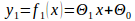Where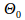and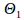are the model parameters &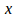is the only feature used.

The plot of the previous model is shown below:Based on a loss function such as the one shown below, we can conclude that the model is not fitting the data well.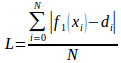Where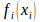is the expected output for sample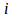and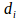is the desired output for the same sample.

The model is too simple and there are many predictions that are not accurate. For such reason, we should create a more complex model that can fit the data well. For such reason, we can increase the degree of the equation from one to two. It will be as follows: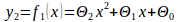By using the same featureafter being raised to power 2 (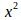), we created a new feature and we will not only capture the linear properties of the data, but also some non-linear properties. The graph of the new model will be as follows:The graph shows that the second degree polynomial fits the data better than the first degree. But also the quadratic equation does not fit well some of the data samples. This is why we can create a more complex model of the third degree with the following equation: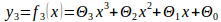The graph will be as follows: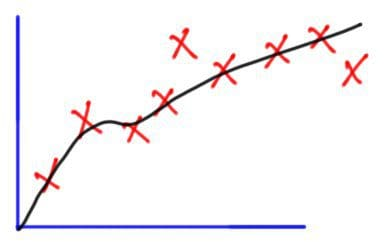It is noted that the model fits the data better after adding a new feature that capturing the data properties of the third degree. To fit the data better than before, we can increase the degree of the equation to be of the fourth degree as in the following equation: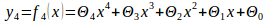The graph will be as follows:It seems that the higher the degree of the polynomial equation the better it fits the data. But there are some important questions to be answered. If increasing the degree of the polynomial equation by adding new features enhances the results, so why not using a very high degree such as 100th degree? What is the best degree to be used for a problem?

Model Capacity/Complexity

There is a term called model capacity or complexity. Model capacity/complexity refers to the level of variation that the model can work with. The higher the capacity the more variation the model can cope with. The first model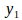is said to be of a small capacity compared to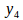. In our case, the capacity increases by increasing the polynomial degree.

For sure the higher the degree of the polynomial equation the more fit it will be for the data. But remember that increasing the polynomial degree increases the complexity of the model. Using a model with a capacity higher than required may lead to overfitting. The model becomes very complex and fits the training data very well but unfortunately, it is a very weak for unseen data. The goal of ML is not only creating a model that is robust with the training data but also to the unseen data samples.
The model of the fourth degree () is very complex. Yes, it fits the seen data well but it will not for unseen data. For such case, the newly used feature inwhich is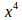captures more details than required. Because that new feature makes the model too complex, we should get rid of it.

In this example, we actually know which features to remove. So, we can remove it and return back to the previous model of the third-degree (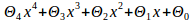). But in actual work, we do not know which features to remove. Moreover, assume that the new feature is not too bad and we do not want to completely remove it and just want to penalize it. What should we do?

Looking back at the loss function, the only goal is to minimize/penalize the prediction error. We can set a new objective to minimize/penalize the effect of the new featureas much as possible. After modifying the loss function to penalize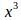, it will be as follows: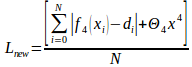Our objective now is to minimize the loss function. We are now just interested in minimizing this term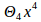. It is obvious that to minimizewe should minimize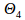as it is the only free parameter we can change. We can set its value to a value equal to zero if we want to remove that feature completely in case it is very bad one as shown below: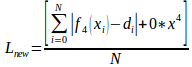By removing it, we go back to the third-degree polynomial equation (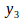).does not fit the seen data perfectly as inbut generally, it will have a better performance for unseen data than.

But in case itis a relatively good feature and we just want to penalize it but not to remove it completely, we can set it to a value close to zero but not to zero (say 0.1) as shown next. By doing that, we limit the effect of. As a result, the new model will not be complex as before.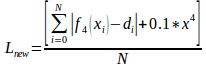Going back to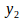, it seems that it is the simpler than. It can work well with both seen and unseen data samples. So, we should remove the new feature used inwhich isor just penalize it if it relatively does well. We can modify the loss function to do that.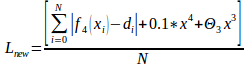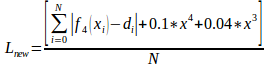Regularization

Note that we actually knew thatis the best model to fit the data because the data graph is available for us. It is a very simple task that we can solve manually. But if such information is not available for us and as the number of samples and data complexity increases, we will not be able to reach such conclusions easily. There must be something automatic to tell us which degree will fit the data and tell us which features to penalize to get the best predictions for unseen data. This is regularization.

Regularization helps us to select the model complexity to fit the data. It is useful to automatically penalize features that make the model too complex. Remember that regularization is useful if the features are not bad and relatively helps us to get good predictions and we just need to penalize but not to remove them completely. Regularization penalizes all used features, not a selected subset. Previously, we penalized just two featuresandnot all features. But it is not the case with regularization.

Using regularization, a new term is added to the loss function to penalize the features so the loss function will be as follows: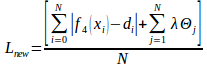It can also be written as follows after moving Λ outside the summation: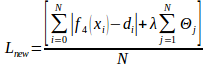The newly added term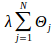is used to penalize the features to control the level of model complexity. Our previous goal before adding the regularization term is to minimize the prediction error as much as possible. Now our goal is to minimize the error but to be careful of not making the model too complex and avoids overfitting.

There is a regularization parameter called lambda (λ) which controls how to penalize the features. It is a hyperparameter with no fixed value. Its value is variable based on the task at hand. As its value increases as there will be high penalization for the features. As a result, the model becomes simpler. When its values decrease there will be a low penalization of the features and thus the model complexity increases. A value of zero means no removal of features at all.

When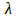is zero, then the values of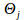will not be penalized at all as shown in the next equation. This is because settingto zero means the removal of the regularization term and just leaving the error term. So, our objective will return back to just minimize the error to be close to zero. When error minimization is the objective, the model may overfit.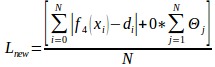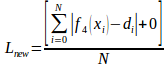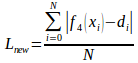But when the value of the penalization parameteris very high (say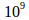), then there must be a very high penalization for the parametersin order to keep the loss at its minimum value. As a result, the parameterswill be zeros. As a result, the model () will have its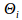pruned as shown below.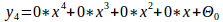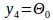Please note that the regularization term starts its index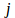from 1 not zero. Actually, we use the regularization term to penalize features (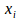). Becausehas not associated feature, then there is no reason to penalize it. In such case, the model will bewith the following graph:Bio: Ahmed Gad received his B.Sc. degree with excellent with honors in information technology from the Faculty of Computers and Information (FCI), Menoufia University, Egypt, in July 2015. For being ranked first in his faculty, he was recommended to work as a teaching assistant in one of the Egyptian institutes in 2015 and then in 2016 to work as a teaching assistant and a researcher in his faculty. His current research interests include deep learning, machine learning, artificial intelligence, digital signal processing, and computer vision.

Original. Reposted with permission.

Related: